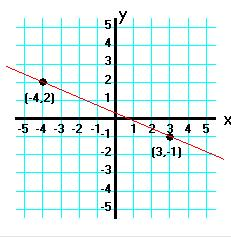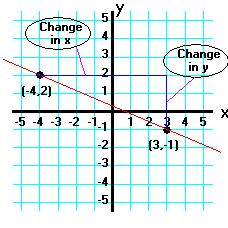# The Slope of a Line

 Our Algebra 1 tutorial software program contains over 60 topic areas. One of them is The Slope of a Line, and this page summarizes the main ideas of this topic. This page is intended for review, and is not a substitute for the interactive, self-paced tutorials of the MathTutor algebra 1 software program.

General Explanation

Look at the line below:
``````
One property of a line is its "steepness." In algebra, steepness is defined by SLOPE.

By definition, slope = change in y/change in x. The change in y and the change in x are shown in the graph below.
``````
Change in y = change in y coordinates = (-1-2) = -3

Change in x = change in x coordinates = (3-(-4)) = 7

So the slope of the line = -3/7

Sample Problem

The slope of the line connecting the points (a,-1) and (-2,-2) is 1/7. What is a?

Solution

First, write out the equation for slope:
```slope = change in y/change in x
```
Next, find the changes in y and x:
```Change in y: -2-(-1) = -2+1 = -1
Change in x: -2-a
```
Use these values in the equation for slope:
```slope = -1/(-2-a) = 1/7
```
Solve for a:
```-7 = -2 - a
a = 5
```